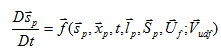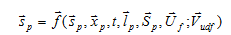# AcuTrace User-Defined Functions Manual

Instructions to define additional solution quantities of AcuTrace called user equations.

AcuTrace is a particle tracer that runs as a post-processor to or a co-processor with AcuSolve. AcuTrace computes particle traces as a series of segments using a fifth-order time-discontinuous Galerkin (TDG) method with error control for solving ordinary differential equations. AcuTrace typically solves for particle position and, optionally, particle stretch.

The user equations are governed either by evolution equations of the form(1)or by evaluation equations of the form(2)where ${\stackrel{⇀}{s}}_{p}$ is the set of user defined particle variables in the current equation, ${\stackrel{⇀}{S}}_{p}$ is the set of user defined variables from the other user equations (if any), ${\stackrel{⇀}{U}}_{f}$ is the set of flow variables, and ${\stackrel{⇀}{V}}_{udf}$ is the set of user equation parameters. User-defined functions are used to define the right hand side of these additional evolution and evaluation equations.

Two steps are needed to use user-defined functions:
• Build a user-defined function
• Reference it in the input file

User-Defined Functions in AcuTrace are referred to as UFPs (for User-Defined Function Particle) to differentiate them from the UDFs used in AcuSolve.

Any number of user-defined functions may be used in a given problem. As further explained below, the user defined functions are compiled and linked into one or more dynamic shared libraries. The script acuMakeLib on Unix/Linux machines and acuMakeDll on Windows machines may be used for this purpose. A list of these libraries is then given to AcuTrace via the configuration option user_libraries. The solver then sequentially searches through these libraries for the user-defined functions referenced in the input file.